Ex 9.1

Chapter 9 Class 10 Some Applications of Trigonometry
Serial order wise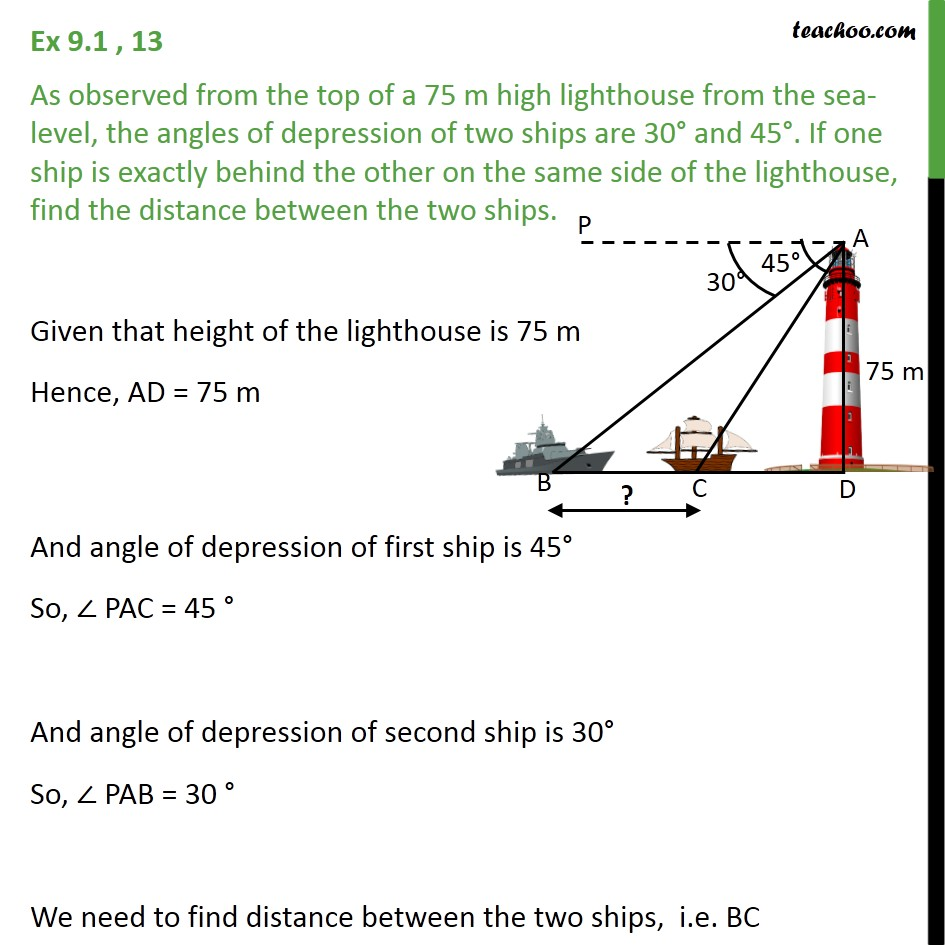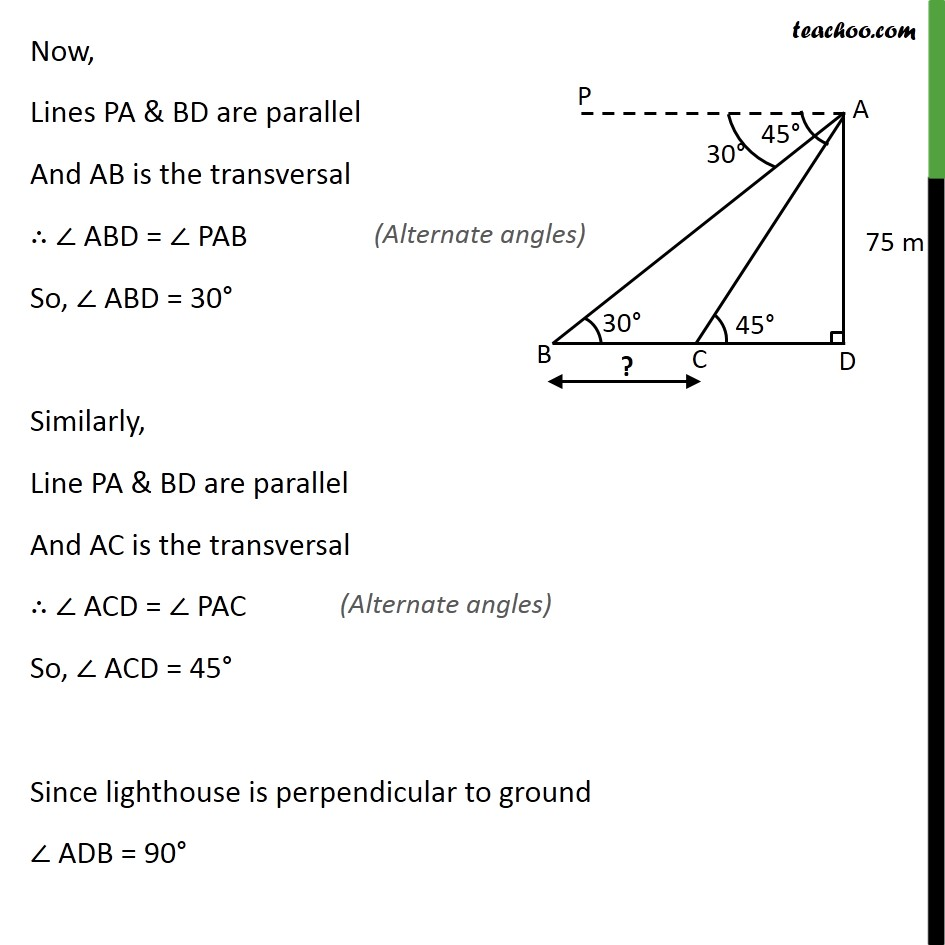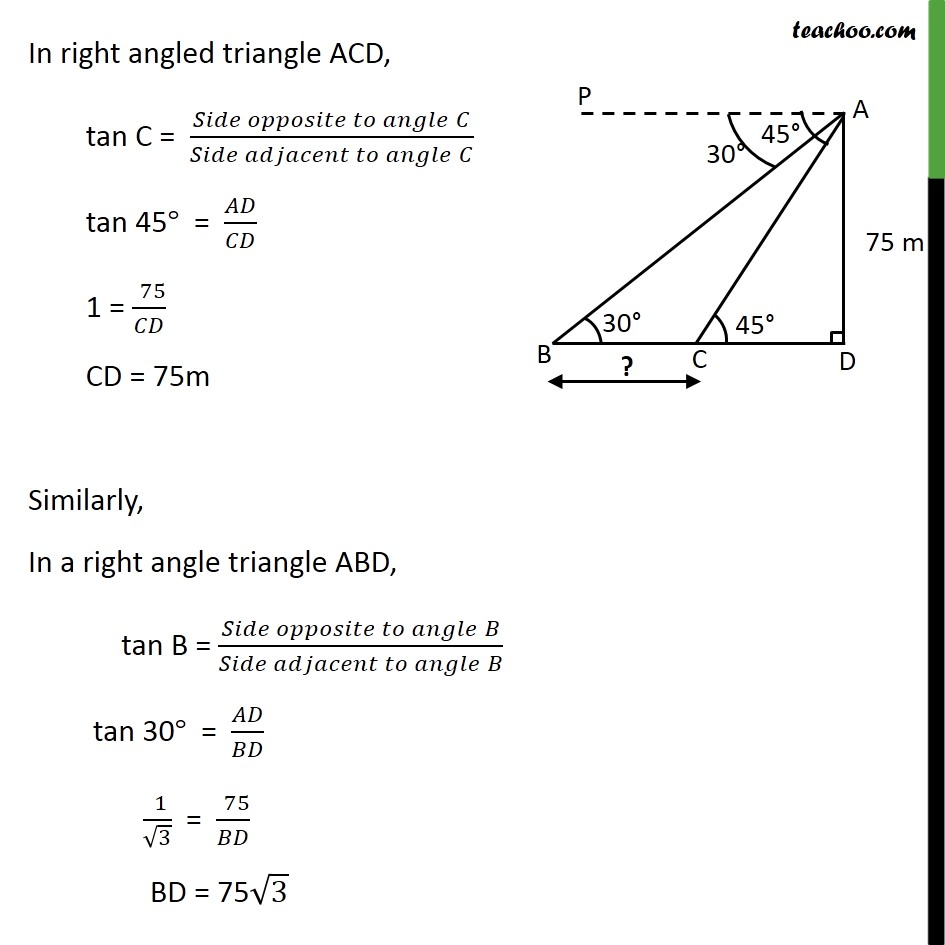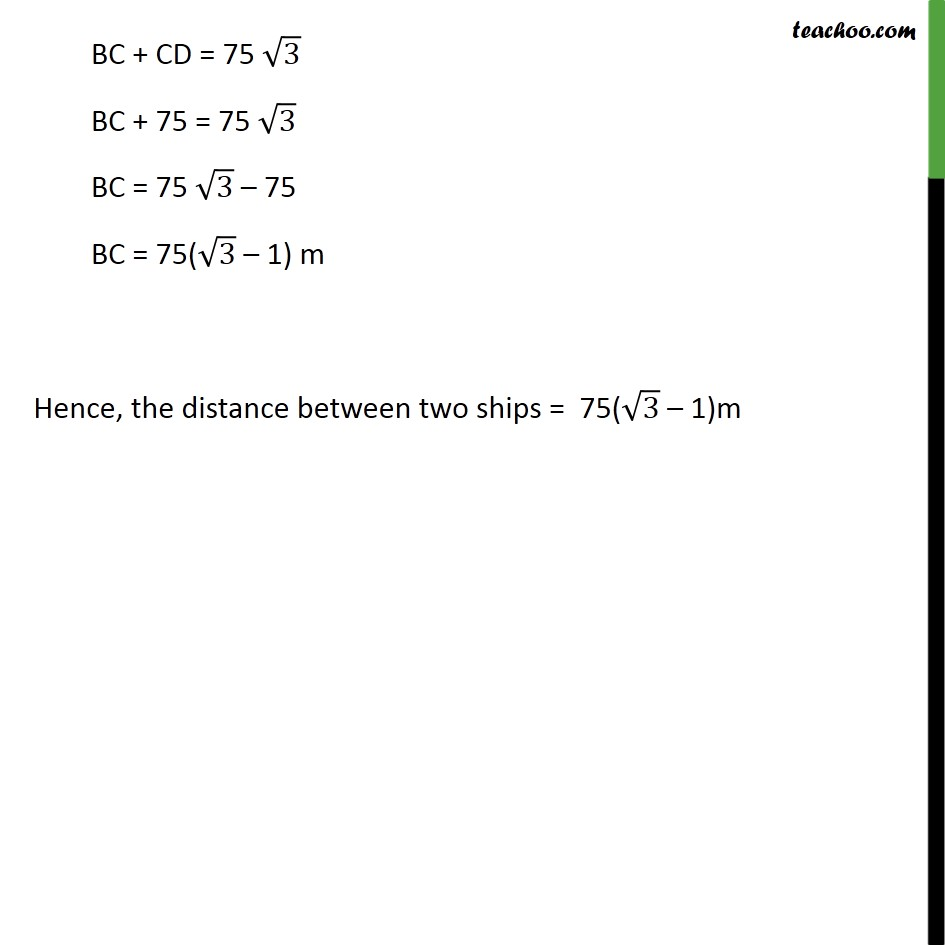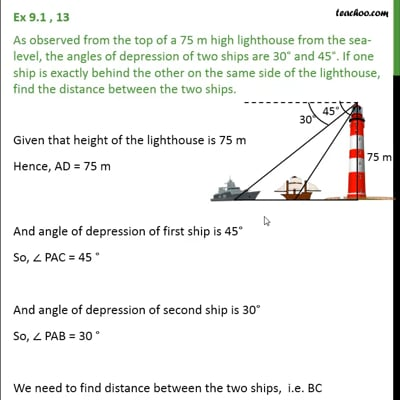This video is only available for Teachoo black users

Solve all your doubts with Teachoo Black (new monthly pack available now!)

### Transcript

Ex 9.1 , 13 As observed from the top of a 75 m high lighthouse from the sea-level, the angles of depression of two ships are 30° and 45°. If one ship is exactly behind the other on the same side of the lighthouse, find the distance between the two ships. Given that height of the lighthouse is 75 m Hence, AD = 75 m And angle of depression of first ship is 45° So, ∠ PAC = 45 ° And angle of depression of second ship is 30° So, ∠ PAB = 30 ° We need to find distance between the two ships, i.e. BC Now, Lines PA & BD are parallel And AB is the transversal ∴ ∠ ABD = ∠ PAB So, ∠ ABD = 30° Similarly, Line PA & BD are parallel And AC is the transversal ∴ ∠ ACD = ∠ PAC So, ∠ ACD = 45° Since lighthouse is perpendicular to ground ∠ ADB = 90° In right angled triangle ACD, tan C = (𝑆𝑖𝑑𝑒 𝑜𝑝𝑝𝑜𝑠𝑖𝑡𝑒 𝑡𝑜 𝑎𝑛𝑔𝑙𝑒" " 𝐶)/(𝑆𝑖𝑑𝑒 𝑎𝑑𝑗𝑎𝑐𝑒𝑛𝑡 𝑡𝑜 𝑎𝑛𝑔𝑙𝑒" " 𝐶) tan 45° = 𝐴𝐷/𝐶𝐷 1 = (" " 75)/𝐶𝐷 CD = 75m Similarly, In a right angle triangle ABD, tan B = (𝑆𝑖𝑑𝑒 𝑜𝑝𝑝𝑜𝑠𝑖𝑡𝑒 𝑡𝑜 𝑎𝑛𝑔𝑙𝑒" " 𝐵)/(𝑆𝑖𝑑𝑒 𝑎𝑑𝑗𝑎𝑐𝑒𝑛𝑡 𝑡𝑜 𝑎𝑛𝑔𝑙𝑒" " 𝐵) tan 30° = 𝐴𝐷/𝐵𝐷 (" " 1)/√3 = (" " 75)/𝐵𝐷 BD = 75√3 BC + CD = 75 √3 BC + 75 = 75 √3 BC = 75 √3 – 75 BC = 75(√3 – 1) m Hence, the distance between two ships = 75(√3 – 1)m